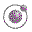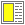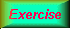Higher-order Functions - slide 16 : 34

The reduction functions

The function reduce-right is a straightforward recursive function

The function reduce-left is a straightforward iterative functionThe function reduce-right.The function reduce-left.
 Expression Value `(reduce-left - '(1 2 3 4 5))` `-13` `(reduce-right - '(1 2 3 4 5))` `3` `(reduce-left string-append (list "The" " " "End"))` `"The End"` ```(reduce-left append (list (list 1 2 3) (list 'a 'b 'c)))``` `(1 2 3 a b c)`Quantifier Functions